One-Sided Fixed-Sample Tests in Clinical Trials

A one-sided test has either an upper (greater) or a lower (lesser) alternative. This section describes one-sided tests with upper alternatives only. Corresponding results for one-sided tests with lower alternatives can be derived similarly.

For a one-sided test of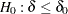with an upper alternative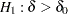, an equivalent null hypothesis is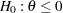with an upper alternative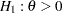, where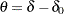. A fixed-sample test rejects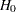if the standardized test statistic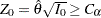, where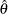is the sample estimate of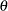and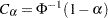is the critical value.

The-value of the test is given by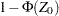, and the hypothesisis rejected if the-value is less than. An upper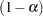confidence interval has the lower limit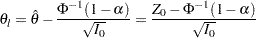The hypothesisis rejected if the confidence interval for the parameterdoes not contain zero—that is, if the lower limit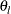is greater than.

With an alternative reference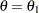,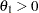, a Type II error probability is defined as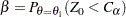which is equivalent to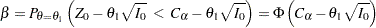Thus,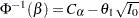. Then, with,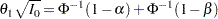The drift parameter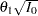can be computed for specifiedandand the maximum information is given by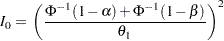If the maximum information is available, then the required sample size can be derived. For example, in a one-sample test for the mean with a specific standard deviation
, the sample sizerequired for the test is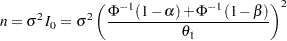On the other hand, if the alternative reference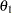, standard deviation, and sample sizeare all specified, thencan be derived for a givenand, similarly,can be derived for a given.

With an alternative reference,, the power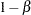is the probability of correctly rejecting the null hypothesisat: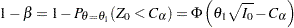### Superiority Trials

A superiority trial that tests the response to a new drug is clinically superior to a comparative placebo control or active control therapy. If a positive value indicates a beneficial effect, a test for superiority has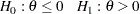whereis the hypothesis of nonsuperiority and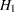is the alternative hypothesis of superiority.

The superiority test rejects the hypothesisand declares superiority if the standardized statistic, where the critical value.

For example, ifis the response difference between the treatment and placebo control groups, then a superiority trial can be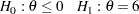with a Type I error probability level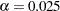and a power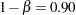at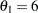.

### Noninferiority Trials

A noninferiority trial does not compare the response to a new treatment with the response to a placebo. Instead, it demonstrates the effectiveness of a new treatment compared with that of a nonexisting placebo by showing that the response of a new treatment is not clinically inferior to the response of a standard therapy with an established effect. That is, this type of trial attempts to demonstrate that the new treatment effect is not worse than the standard therapy effect by an acceptable margin. These trials are often performed when there is an existing effective therapy for a serious disease, and therefore a placebo control group cannot be ethically included.

It can be difficult to specify an appropriate noninferiority margin. One practice is to choose with reference to the effect of the active control in historical placebo-controlled trials (Snapinn 2000, p. 20). With this practice, there is some basis to imply that the new treatment is better than the placebo for a positive noninferiority trial.

If a positive value indicates a beneficial effect, a test for noninferiority has a null hypothesis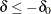and an alternative hypothesis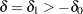, where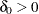is the specified noninferiority margin.

An equivalent test has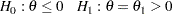where the parameter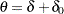,is the null hypothesis of inferiority, andis the alternative hypothesis of noninferiority,

The noninferiority test rejects the hypothesisand declares noninferiority if the standardized statistic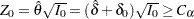, where the critical value.

For example, if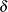is the response difference between the treatment and active control groups and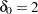is the noninferiority margin, then a noninferiority trial with a powerat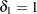might be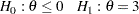where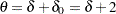.